##### Linear Algebra For Dummies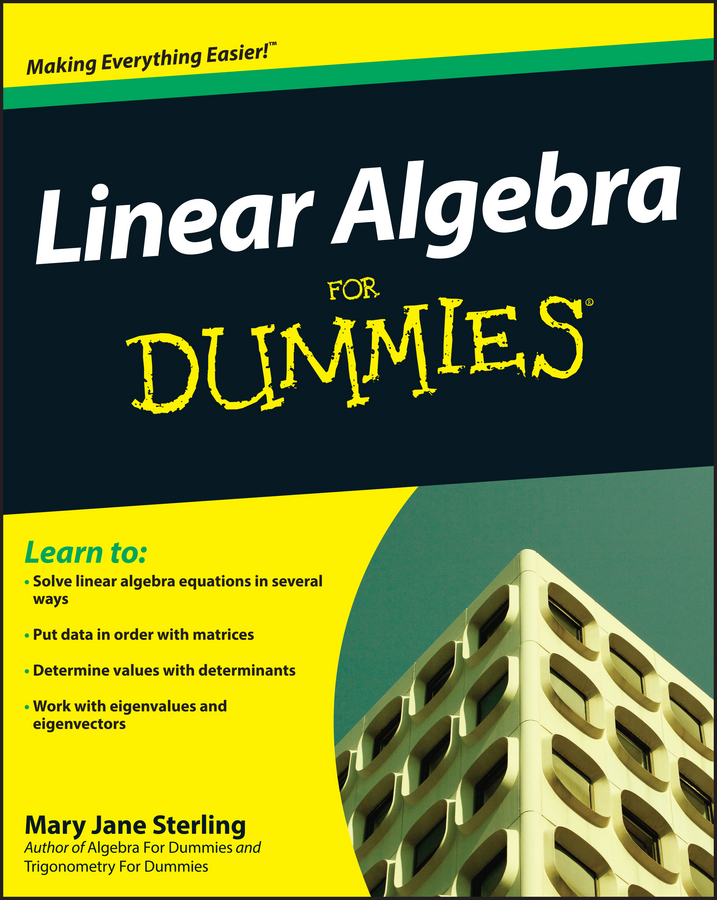A convention of mathematics is that you don’t leave radicals in the denominator of an expression when you write it in its final form. Thus we do something called rationalizing the denominator. This convention makes collecting like terms easy, and your answers will be truly simplified.

A numerator can contain a radical, but the denominator can’t. The final expression may look more complicated in its rational form, but that’s what you have to do sometimes.

There are two separate situations where radicals may show up in the denominator of a fraction: where expressions contain one radical in the denominator, and where expressions contain two terms in the denominator, at least one of which is a radical.

## Rationalizing with one radical in the denominator

Rationalizing expressions with one radical in the denominator is easy. For example, with a square root, you just need to get rid of the square root. Normally, the best way to do that in an equation is to square both sides. For example,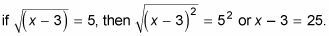However, you can’t fall for the trap of rationalizing a fraction by squaring the numerator and the denominator. For instance, squaring the top and bottom of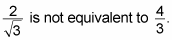1. Multiply the numerator and the denominator by the same square root.

Whatever you multiply to the bottom of a fraction, you must multiply to the top; this way, it’s really like you multiplied by one and you didn’t change the fraction. Here’s what it looks like: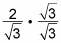2. Multiply the tops and multiply the bottoms and simplify.

For this example, you get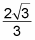The process for rationalizing a cube root in the denominator is quite similar to that of rationalizing a square root. To get rid of a cube root in the denominator of a fraction, you must cube it. If the denominator is a cube root to the first power, for example, you multiply both the numerator and the denominator by the cube root to the 2nd power to get the cube root to the 3rd power (in the denominator). Raising a cube root to the 3rd power cancels the root — and you’re done!

## Rationalizing when the denominator is a binomial with at least one radical

You must rationalize the denominator of a fraction when it contains a binomial with a radical. For example, look at the following equations: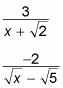Getting rid of the radical in these denominators involves using the conjugate of the denominators. A conjugate is a binomial formed by taking the opposite of the second term of the original binomial. The conjugate of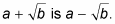The conjugate of x + 2 is x – 2; similarly, the conjugate of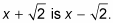Multiplying a number by its conjugate is really the FOIL method in disguise. Remember from algebra that FOIL stands for first, outside, inside, and last.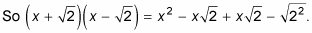The middle two terms always cancel each other, and the radicals disappear. For this problem, you get x2 – 2.

Take a look at a typical example involving rationalizing a denominator by using the conjugate. First, simplify this expression: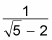To rationalize this denominator, you multiply the top and bottom by the conjugate of it, which is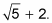The step-by-step breakdown when you do this multiplication is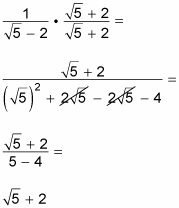Here’s a second example: Suppose you need to simplify the following problem: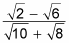1. Multiply by the conjugate.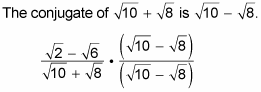2. Multiply the numerators and denominators.

FOIL the top and the bottom. (Tricky!) Here’s how you do it: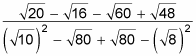3. Simplify.

Both the numerator and denominator simplify first to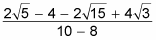which becomes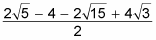This expression simplifies even further because the denominator divides into every term in the numerator, which gives you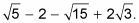Simplify any radical in your final answer — always. For example, to simplify a square root, find perfect square root factors: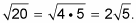Also, you can add and subtract only radicals that are like terms. This means the number inside the radical and the index (which is what tells you whether it’s a square root, a cube root, a fourth root, or whatever) are the same.